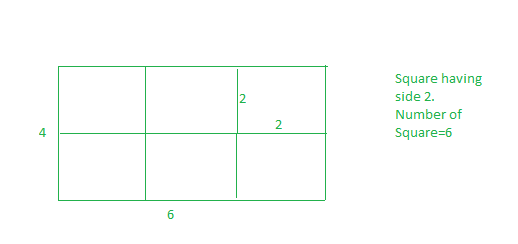Related Articles
Minimum squares to evenly cut a rectangle
• Difficulty Level : Easy
• Last Updated : 28 Nov, 2018

Given a rectangular sheet of length l and width w. we need to divide this sheet in the square sheets such that the number of square sheets should be as minimum as possible.

Examples:

Input :l= 4 w=6
Output :6
We can form squares with side of 1 unit, But the number of squares will be 24, this is not minimum. If we make square with side of 2, then we have 6 squares. and this is our required answer.
And also we can’t make square with side 3, if we select 3 as square side, then whole sheet can’t be converted into suares of equal length.Input :l=3 w=5
Output :15

## Recommended: Please try your approach on {IDE} first, before moving on to the solution.

Optimal length of side of a square is equal to GCD of two numbers

## C++

 `// CPP program to find minimum number of``// squares to make a given rectangle.``#include ``using` `namespace` `std;`` ` `int` `countRectangles(``int` `l, ``int` `w)``{``    ``// if we take gcd(l, w), this``    ``// will be largest possible``    ``// side for suare, hence minimum``    ``// number of square.``    ``int` `squareSide = __gcd(l, w);`` ` `    ``// Number of squares.``    ``return` `(l * w) / (squareSide * squareSide);``}`` ` `// Driver code``int` `main()``{``    ``int` `l = 4, w = 6;``    ``cout << countRectangles(l, w) << endl;``    ``return` `0;``}`

## Java

 `// Java program to find minimum number of``// squares to make a given rectangle.`` ` `class` `GFG{``static` `int` `__gcd(``int` `a, ``int` `b) {``   ``if` `(b==``0``) ``return` `a;``   ``return` `__gcd(b,a%b);``}``static` `int` `countRectangles(``int` `l, ``int` `w)``{``    ``// if we take gcd(l, w), this``    ``// will be largest possible``    ``// side for suare, hence minimum``    ``// number of square.``    ``int` `squareSide = __gcd(l, w);`` ` `    ``// Number of squares.``    ``return` `(l * w) / (squareSide * squareSide);``}`` ` `// Driver code``public` `static` `void` `main(String[] args)``{``    ``int` `l = ``4``, w = ``6``;``    ``System.out.println(countRectangles(l, w));``}``}``// This code is contributed by mits`

## Python3

 `# Python3 code to find minimum number of``# squares to make a given rectangle.`` ` `import` `math `` ` `def` `countRectangles(l, w):`` ` `    ``# if we take gcd(l, w), this``    ``# will be largest possible``    ``# side for suare, hence minimum``    ``# number of square.``    ``squareSide ``=` `math.gcd(l,w)``     ` `    ``# Number of squares.``    ``return` `(l``*``w)``/``(squareSide``*``squareSide)`` ` `# Driver Code``         ` `if` `__name__ ``=``=` `'__main__'``:``    ``l ``=` `4``    ``w ``=` `6``    ``ans ``=` `countRectangles(l, w)``    ``print` `(``int``(ans))`` ` `# this code is contributed by``# SURENDRA_GANGWAR`

## C#

 `// C# program to find minimum number of``// squares to make a given rectangle.`` ` `class` `GFG{``static` `int` `__gcd(``int` `a, ``int` `b) {``if` `(b==0) ``return` `a;``return` `__gcd(b,a%b);``}``static` `int` `countRectangles(``int` `l, ``int` `w)``{``    ``// if we take gcd(l, w), this``    ``// will be largest possible``    ``// side for suare, hence minimum``    ``// number of square.``    ``int` `squareSide = __gcd(l, w);`` ` `    ``// Number of squares.``    ``return` `(l * w) / (squareSide * squareSide);``}`` ` `// Driver code``public` `static` `void` `Main()``{``    ``int` `l = 4, w = 6;``    ``System.Console.WriteLine(countRectangles(l, w));``}``}``// This code is contributed by mits`

## PHP

 ``
Output:
```6
```

Attention reader! Don’t stop learning now. Get hold of all the important DSA concepts with the DSA Self Paced Course at a student-friendly price and become industry ready.

My Personal Notes arrow_drop_up